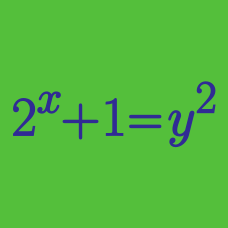Algebra

# Algebraic Manipulation - Identities

If $x$ and $y$ are positive integers satisfying the equation $3x+5y=90$, what is the maximum possible value of $x+y$?

If the following equality always holds regardless of the value of $k$, what is $x+y+z$? $(k^2+k)x-(k^2-k)y-(k-3)z=30?$

If positive integers $x$ and $y$ satisfy the equation $xy-5x-10y+37=0$, the sum $x+y$ is constant. What is the value of $x+y$?

If $a+b+c=1, a^2+b^2+c^2=11,$ and $abc=2,$ what is the value of $a^2b^2+b^2c^2+c^2a^2?$

If $x^8 = 829$, what is $(x-1)(x+1)(x^2+1)(x^4+1)$?

×